up

# Physical Basics of Quantum Computing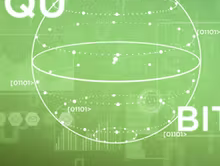Start date will be announced later
• English

course language

• 6 weeks

course duration

• от 4 до 6 часов в неделю

needed to educate

• 2 credit points

Quantum information and quantum computations is a new, rapidly developing branch of physics that has arisen from quantum mechanics, mathematical physics and classical information theory. Significant interest in this area is explained by the great prospects that will open upon the implementation of its ideas, capturing almost all areas of human activity related to the transfer, storage and processing of information.
The purpose of this course is to show the basic ideas of quantum informatics, as well as the physical laws and basic mathematical principles. Much attention is paid to such phenomena as quantum entanglement, quantum parallelism, and quantum interference. It is these phenomena that underlie most of the known quantum protocols and algorithms, which are devoted to individual sections of this course. In particular, from the course, students will learn about quantum teleportation, quantum algorithms, quantum error correction and other topics related to the quantum computations theory.
As a result of the course, the students will be able to master the modern mathematical apparatus of quantum mechanics used in quantum computations, master the ideas that underlie the most important quantum logic algorithms and protocols for transmitting and processing quantum information, and learn how to solve problems on these topics.

The course consists of six modules, which are arranged in two parts. The first part (Modules 1-2) focuses on the mathematical apparatus and the foundations of Quantum Mechanics. Physical laws and processes which underlay quantum computations are extensively described. In the second part of the course (Modules 3-6), we show how quantum computations, quantum logic algorithms and protocols of quantum information transfer could be implemented using the laws of quantum physics and phenomena discussed in the first part.
Module 1 focuses on the postulates of Quantum Mechanics and Quantum Information Theory. We introduce the important concept of a qubit and consider variants of its physical implementation. Certain statistical aspects of quantum theory are discussed. The concept of density matrix and separability, the notion of pure and mixed quantum states are introduced. In Module 2 we focus on the phenomenon of quantum entanglement and the mathematical description of entangled physical systems. We also describe an experiment aimed to test Bell inequalities and consider the well-known EPR paradox. In Module 3 we compare classical and quantum computations. Particularly, elementary logical elements (gates) and the simplest commutation schemes are described. The distinctive features of quantum computations are explained in Module 4. In particular, the No-Cloning theorem is proved, which forbids one to copy a qubit, quantum parallelism and superdense coding are discussed. We also describe in detail the protocol of quantum teleportation and give an example of its physical implementation. The well-known quantum logic algorithms are described in Module 5, these are Deutsch and Deutsch-Jozsa algorithms, Quantum Fourier Transform and Shor’s algorithms for integer factorization. In Module 6 we discuss quantum and classical error correction theory: we highlight their differences and similarities, classify the possible error types and describe protocols of their correction.
The material is given in a form of short but informative videos with presentations and a detailed syllabus. At the end of every module, there are tests and control tasks according to the discussed topics. The tasks are organized in the form of problems and tests with multiple choice. Every module is supplemented with a literature list, which contains references on the mentioned researches and extra sources for self-study.

## Format

The material is presented in the form of short but informative videos with presentations and detailed summaries. At the end of each module, verification and control tasks on the topics covered. Assignments are presented in the form of multiple choice tests and tasks. List of references for each module contains both the studies mentioned in the course and additional sources for independent study.

## Course program

Program
Module 1. Statistical aspects of quantum mechanics. Qubit. Physical implementation of a qubit. Qubits as a quantum unit of information. Bloch Sphere. Pure and mixed states of quantum systems. Density matrix and its properties. Qubit systems. Inseparability of quantum systems. The reduced density matrix.
Module 2. Quantum entanglement. Schmidt decomposition. Bellstates. EPR paradox. Bell inequalities.
Module 3. Classical and quantum logical operations. General principles of classical computations. The simplest classical computations. Landauer principle. Reversible gates. Pauli matrices. Single-qubit logic gates. Controlled quantum logic gates.
Module 4. Distinctivefeaturesofquantumcomputations. No-cloningtheorem. Superdense coding. Quantumteleportation. Experimentonqubitquantumteleportation. Quantumparallelism.
Module 5. Quantum Algorithms. Deutsch algorithm. Deutsch–Jozsa algorithm. Quantum Fourier Transform. Eigenvalue algorithm. Shor's algorithm for integer factorization.
Module 6. Basics of error correction theory. Distinctive features of classical error correction theory. Classical three-bit code. Distinctive features of quantum error correction theory. Three-qubit code.

## Education results

Learning outcomes
The student who completed this course should:
To know
•    fundamental concepts of quantum mechanics and the quantum information theory;
•    The most important protocols for the transfer and processing of quantum information;
•    The most important quantum logic algorithms;
•    The basic protocols of the classical and quantum error correction theory.
To be able to
•    work with classical and quantum circuits;
•    solve problems in the quantum information theory.
To master
•    mathematical apparatus of quantum mechanics used in the quantum information theory.

## Knowledge

<pre>fundamental concepts of quantum mechanics and quantum information theory; the most important protocols for the transmission and processing of quantum information; the most important quantum logic algorithms; basic protocols of classical and quantum error theory.</pre>

## Skills

<pre>mathematical apparatus of quantum mechanics, used in the theory of quantum information.</pre>

## Abilities

<pre>work with classical and quantum switching circuits; solve problems in quantum information theory</pre>

The course is aimed at the formation of general learning competencies for Bachelor’s and Specialists’ programs, as well as other competencies included in the education program.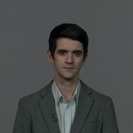### Тихонов Кирилл Сергеевич

Кандидат физико-математических наук
Position: старший преподаватель кафедры Общей физики-1, Лаборатория Квантовой Оптики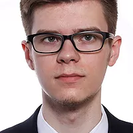### Выборный Иван Михайлович

Position: студент физического факультета СПбГУ, кафедра Общей физики-1, Лаборатория Квантовой Оптики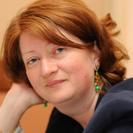### Голубева Татьяна Юрьевна

Доктор физико-математических наук
Position: профессор кафедры Общей физики-1, Лаборатория Квантовой Оптики### Вашукевич Евгений Александрович

Position: аспирант физического факультета СПбГУ, кафедра Общей физики-1, Лаборатория Квантовой Оптики

## Similar courses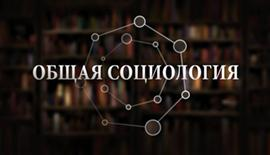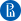НИУ ВШЭ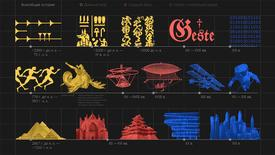СПбГУ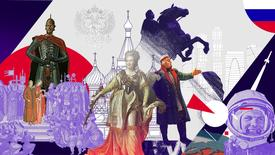СПбГУ